# How to Calculate Sheet Metal Unfold With K Factor?

In recent years, the sheet metal bending process, as an important processing method, has developed rapidly in the field of rail transit.

As a comprehensive cold working process, the accuracy of the bending development dimension is very important for sheet metal processing enterprises.

Through theoretical analysis of 90 ° bent sheet metal parts, this post deduces the calculation method of K factor and explains the application scope of this calculation method, which provides a theoretical basis and practical reference for engineers and technicians in the sheet metal industry.

## Calculation of K factor for sheet metal bending

In the bending process, the outer layer is subjected to tensile stress and the inner layer is subjected to compressive stress.

There is a transition layer between the outer layer and the inner layer, which is neither subject to tensile stress nor compressive stress.

This transition layer is called the neutral layer. The length of the neutral layer remains unchanged before and after bending, so the length of the neutral layer is calculated when calculating the unfolding size of the sheet metal.

Fig. 1 is a schematic diagram of sheet metal bending dimensions.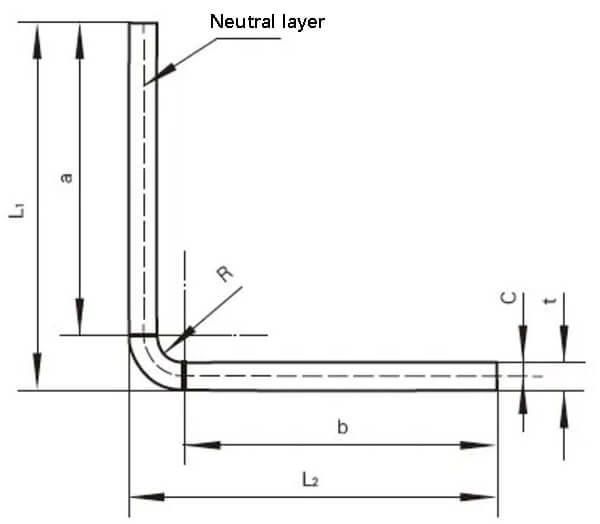Fig. 1 Schematic diagram of sheet metal bending size

As shown in Fig. 1, the unfolding size of the sheet metal is set as L, so there is:

L=a+b+2π(R+C)/t    ①

L1=a+R+t    ②
L2=b+R+t    ③

Where K factor: 0<K=ｃ/ｔ<1　　④

Derived from equations ① ~ ④, it can be obtained that:

K=2(L-L1-L2+2R+2t)/πt – R/t      ⑤

From the calculation results of equation ⑤, it can be seen that the value of the K factor depends on the overall dimension, bending inner diameter and material thickness of sheet metal parts.

## Traditional manual sheet metal unfolding calculation

In the traditional calculation, sheet metal technicians carry out CAD sheet metal unfolding drawing based on the bending coefficient summarized from many years of bending experience, draw the unfolded sheet metal shape, export the DXF format, and then input it into the laser cutting machine for the operation to obtain the sheet metal unfolding shape of the part.

In the traditional manual sheet metal unfolding calculation, the bending coefficient is different in different processing plants.

As shown in Fig. 2, the back cover of a power cabinet of an electric locomotive is made of 1.5mm thick cold-rolled steel plate.

A processing plant calculates its expansion size as follows: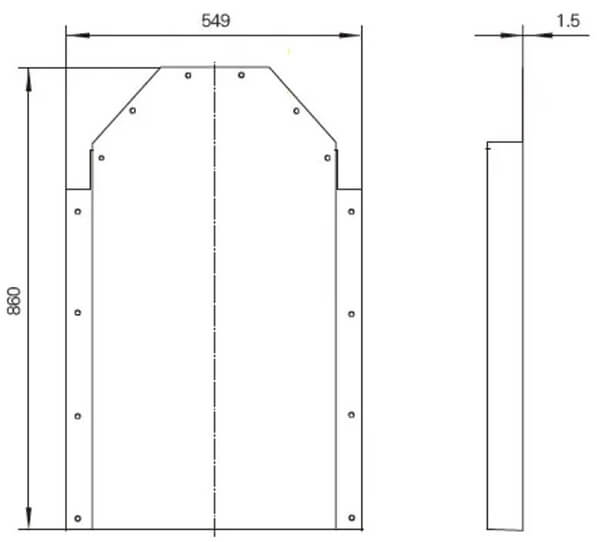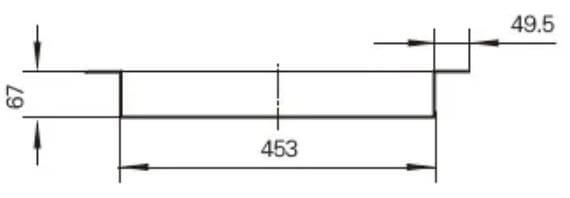Fig. 2 Dimension diagram of rear cover of a power cabinet

Total width = 453 + 67 × 2+49.5 × 2-8 × 1.5 (material thickness) + 4 × 0.5 (bending factor) = 676mm

Total length = 860 + 67-2 × 1.5 (material thickness) + 0.5 (bending coefficient) = 924.5mm

## Calculation of sheet metal unfolding through 3D modeling software by K-Factor method and its application range

The efficiency of manual drawing is low.

The calculation efficiency of sheet metal unfolding is greatly improved by using the three-dimensional modeling software K-factor method.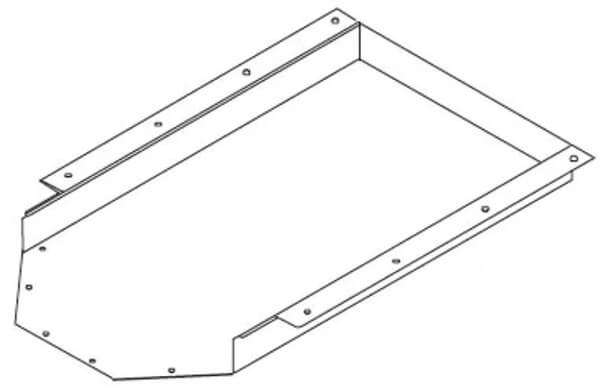Fig. 3 3D view of the back cover of a power cabinet

Based on the traditional manual calculation of the unfolded size of sheet metal, the unfolded size and bending inner diameter are substituted into equation ⑤ to obtain the corresponding K factor.

In the process of sheet metal bending, the smaller the bending inner diameter, the more serious the compression and tension on the inner and outer sides of the material.

When the yield strength of the material is exceeded, cracks and fractures will occur.

For the rear cover of a power cabinet of the electric locomotive in Fig. 2, the bending inner diameter can be selected as 1.5mm in the three-dimensional modeling software, and the K factor calculated by formula ⑤ is 0.486.

For the thickness of other specifications, the K factor can be calculated similarly.

Table 1 shows the bending parameters of a sheet metal processing enterprise.

Table 1 SolidWorks bending parameters

 Material thickness (mm) K factor Bending inner diameter (mm) 1.5 0.486 1.5 2 0.486 2 3 0.486 3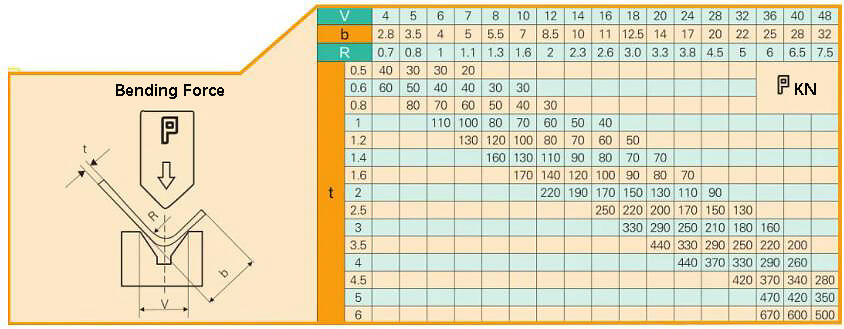Fig. 4 Quick check table of the bending force of press brake machine

The value calculated by the K factor can be input into the 3D modeling software.

When the 3D modeling software is used for direct expansion, there are sometimes some gaps in the expanded drawing, such as local enlarged drawings I and II in Fig. 5.

These notches need to be removed and modified to meet the appearance of laser cutting, as shown in Fig. 6.

DXF drawings exported by 3D modeling software can have bending lines to facilitate subsequent bending.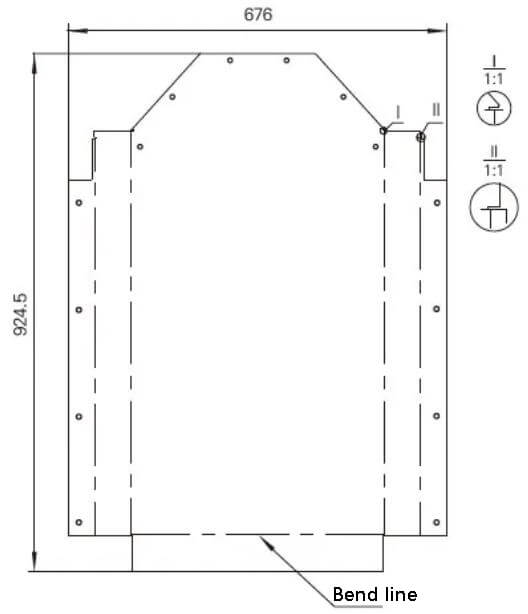Fig. 5 Expanded view of the rear cover of power cabinet directly exported by 3D modeling software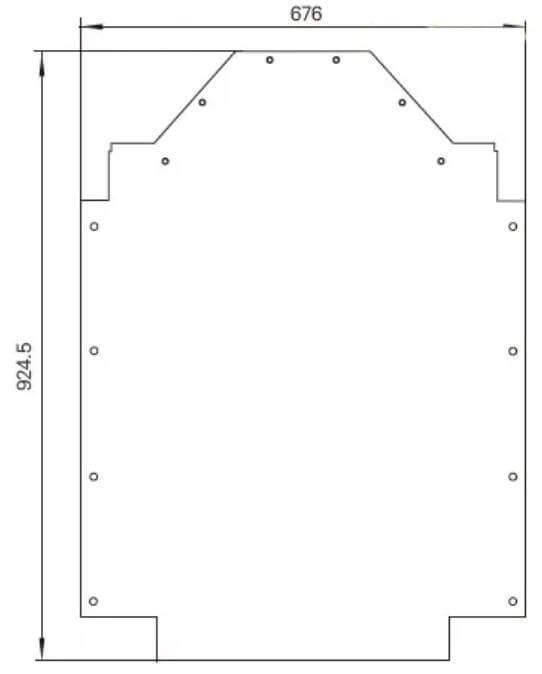Fig. 6 Modified expanded view

## Analysis of bending process

The form of bending tools is shown in Fig. 7.

During processing, appropriate tools are mainly selected according to the shape of the workpiece.

Generally, there are many bending tools in processing enterprises, especially those with a high degree of specialization.

In order to bend various complex sheet metal parts, many bending tools of shapes and specifications are customized.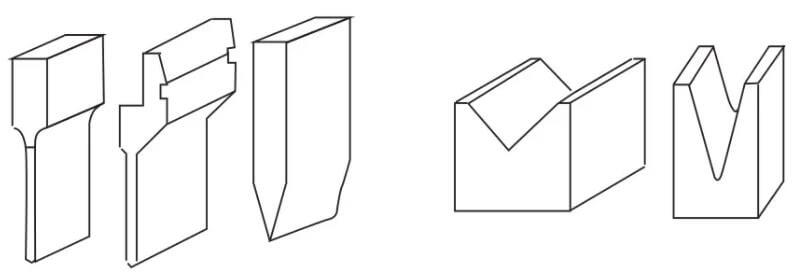Fig. 7 Bending tool

There are many factors affecting the bending process, mainly including the arc radius of the upper die, material, material thickness, strength of the lower die, die size of the lower die and so on.

In order to meet the needs of products and ensure the safety of the bending machine, the sheet metal processing enterprises have serialized the bending die.

We need to have a general understanding of the existing bending die in the process of structural design.

As shown in Fig. 7, the left side is the upper mold and the right side is the lower mold.

The basic principle of bending is to use the bending knife (upper die) and V-groove (lower die) of the bending machine to bend and form the sheet metal parts.

Bending accuracy:

One fold: ± 0.1mm

Two fold: ± 0.2mm

Three fold: ± 0.3mm

and so on.

## Conclusion

In the calculation of sheet metal unfolding by three-dimensional modeling software, the K-factor method can directly export the unfolding drawings with high precision, which is convenient for sheet metal unfolding technicians to no longer redraw the unfolding drawings, improve the processing efficiency of sheet metal production enterprises and shorten the delivery cycle.

## Expert Help and Customized Price Quotes

Need a price quote or have questions? Contact us and let us know your detailed requirements. Our experts will provide you with personalized assistance and a competitive price quote.